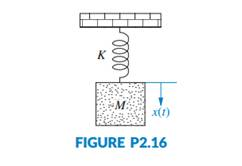### Create an Account

Already have account?

### Forgot Your Password ?

Home / Questions / For the unexcited no external force applied system of Figure P216 do the following a Writ...

# For the unexcited no external force applied system of Figure P216 do the following a Write the differential equation that describes the system b Assuming initial conditions

For the unexcited (no external force applied) system of Figure P2.16, do the following:

a. Write the differential equation that describes the system.

b. Assuming initial conditions x (0) = x0 and ? (0) = x1, write a Laplace transform expression for X(s).

c. Find x(t) by obtaining the inverse Laplace transform from the result in Part c.

d. What will be the oscillation frequency in Hz for this system?Jun 28 2020 View more View Less

#### Answer (Solved)Subscribe To Get Solution